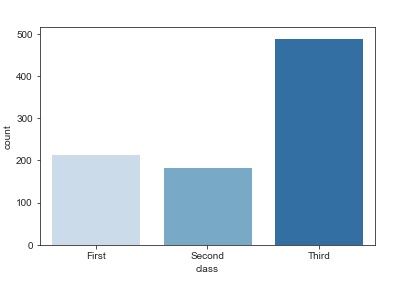# How can the countplot be used to visualize data in Python Seaborn Library?

PythonServer Side ProgrammingProgramming

Seaborn is a library that helps in visualizing data. It comes with customized themes and a high-level interface.

In previous plots, we plotted the entire dataset on the graph. With the help of bar plots, we can understand the central tendency of the distribution of data.

The barplot function establishes the relationship between a categorical variable and a continuous variable. Data is represented in the form of rectangular bars where the length of the bar indicates the proportion of data in that specific category.

A special case of barplot is the countplot that shows the number of observations in every category with respect to the data instead of computing a statistical value of a second variable.

Let us understand the countplot with the help of ‘titanic’ dataset −

## Example

import pandas as pd
import seaborn as sb
from matplotlib import pyplot as plt
sb.countplot(x = "class", data = my_df, palette = "Blues");
plt.show()

## Output## Explanation

• The required packages are imported.
• The input data is ‘titanic’ which is loaded from the seaborn library.
• This data is stored in a dataframe.